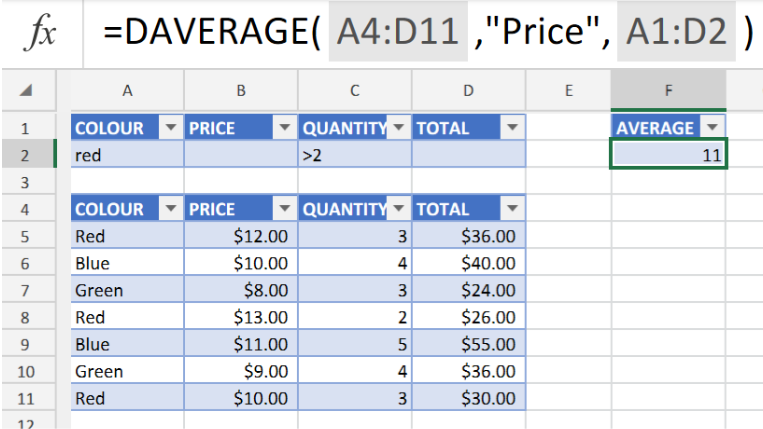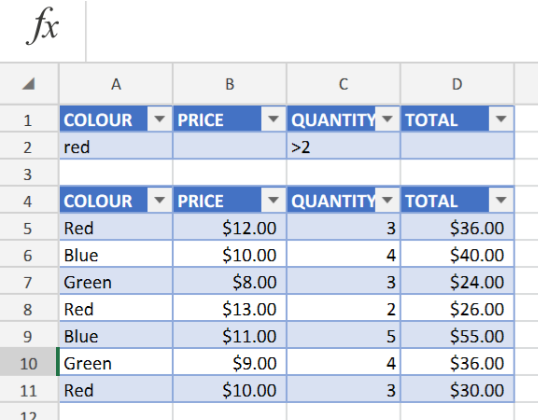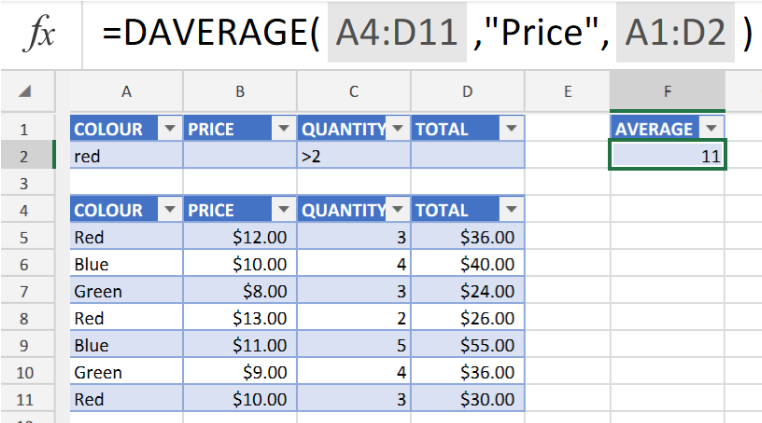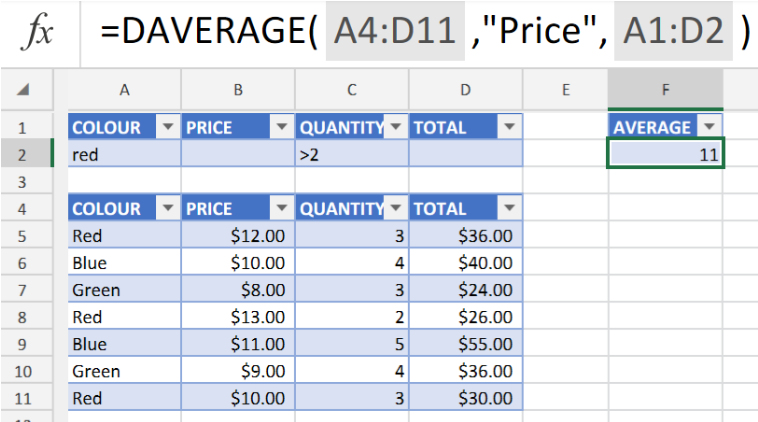Get instant live expert help with Excel or Google Sheets“My Excelchat expert helped me in less than 20 minutes, saving me what would have been 5 hours of work!”

#### Post your problem and you’ll get expert help in seconds.

Your message must be at least 40 characters
Our professional experts are available now. Your privacy is guaranteed.

# Excel DAVERAGE FunctionFigure 1. of the Excel DAVERAGE Function.

The DAVERAGE Function in Excel is used to determine the average amount of data records within a range of data that matches a specific criteria. This tutorial will step through how to use the DAVERAGE function.

## Generic Formula

`DAVERAGE(database, field, criteria)`

We are going to describe and demonstrate the usage and formula syntax in this post.

The Excel DAVERAGE formula syntax has the following components;

• database – our cell range which contains the database information. Basically a list of related information records.
• field – the specific index to be counted.
• criteria – our whole range of criteria (headers inclusive).

How to use the Excel DAVERAGE Function.

Let’s say we have to determine the average in a specific database for a different subset of data records that matches with our criteria.

We can achieve this by following 2 simple steps;

1. Collate and arrange our data values in our Excel sheet. Create separate Tables for our database Values and the field Index to be counted.Figure 2. of Database and Field Entry Tables in Excel.

1. Using the data values in the example illustrated above, we can determine our average price value from the column labeled “Price”, entries where the colours are red and the quantity is less than 2. We will enter the following formula syntax into the cell of a separate column labeled as”AVERAGE” . The cell in our example illustrated below is F2.

`=DAVERAGE(A4:D11,"Price",A1:D2)`Figure 3. of DAVERAGE Function in Excel.

## Note

• The field component of the formula syntax may be given in the form of a name value within double quotes. In our example illustrated above we have used “Price” in our formula syntax.
• Our criteria and database Table range have to be labeled with similar headers.

The DAVERAGE Function in Excel can be categorized as a Database Function. It can be factored in as a component part of an operation syntax entered into a cell in our worksheet.Figure 4. of Final Result.

## Instant Connection to an Expert through our Excelchat Service:

Our live Excelchat Service is here for you. We have Excel Experts available 24/7 to answer any Excel questions you may have. Guaranteed connection within 30 seconds and a customized solution for you within 20 minutes.

Solution examplesHow do I calculate the average for a group of data that is referenced to multiple cells? I.e. If I wanted to know the average salary of 10 employees with the same salary grade but in different departments.
Solved by O. J. in 40 mins